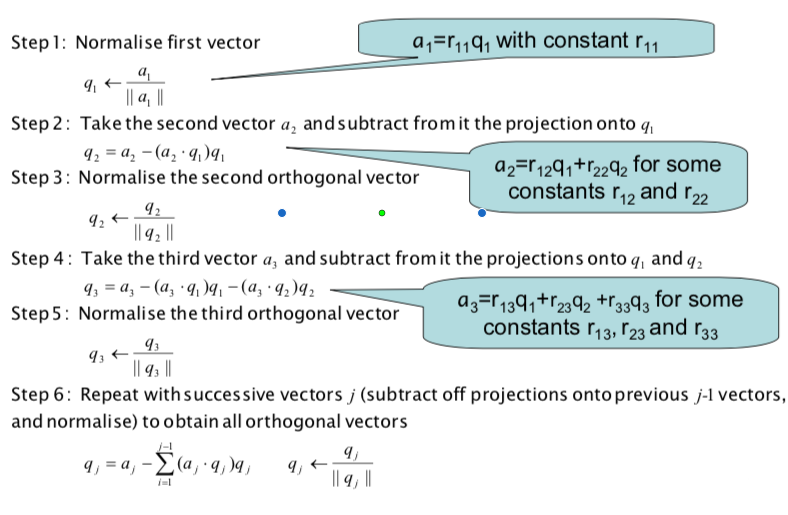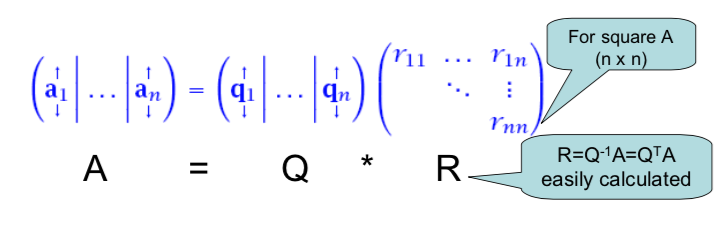School of Economics and Management
Beihang University
http://yanfei.site

## Finding eigenvalues and eigenvectors

• So far, we have only considered the characteristic polynomial approach to find the eigenvalues of a matrix
• Once we have the eigenvalues, we have been solving the homogeneous equation to find the corresponding eigenvectors
• The process is: find the eigenvalues first, and then find the corresponding eigenvectors

## Practicalities

• Unfortunately, this is an impractical approach for $$n > 4$$
• We will bypass the characteristic polynomial and now take a different approach
• The Power Method
• QR decomposition

## The Power Method

• The Power Method finds the dominant eigenvalue $$\lambda_1$$ and corresponding dominant eigenvector $$v_1$$ of a matrix $$A$$
• The dominant eigenvalue is the one with the largest modulus (absolute value for real eigenvalues)
• The Power Method is an iterative approach that generates a sequence of scalars that converge to $$\lambda_1$$ and a sequence of vectors that converge to $$v_1$$
• The Power Method works well (converges quickly) when the dominant eigenvalue is clearly dominant

## The Power Method

• It works by starting with an initial vector $$x_0$$, transforming to $$x_1 = Ax_0$$, transforming $$x_1$$ to $$x_2 = Ax_1$$, etc.

$x_1 = Ax_0;~x_2 = Ax_1 = A^2x_0; \cdots; x_k = A^kx_0.$ - As $$k\rightarrow \infty$$, $$x_k \rightarrow v_1$$.

## Proof of convergence

• Assume $$\lambda_1$$ is the dominant eigenvalue.
• $$|\lambda_1| > |\lambda_2| > \cdots > |\lambda_n|$$.
• Assume there are $$n$$ independent eigenvectors $$v_1, \cdots, v_n$$.
• $$x_0 = c_1 v_1 + \cdots + c_nv_n$$.
• $$x_k = ?$$

## Estimation of eigenvalues

So once we have an eigenvector estimate we can quickly estimate the corresponding eigenvalue

$Ax = \lambda x \Rightarrow x^T Ax = \lambda x^Tx \Rightarrow \lambda = \frac{x^TAx}{x^Tx} = \frac{x^TAx}{||x||^2} = q^TAq.$

## Practical Power method (normalised)

Since the components of $$x_k$$ just get larger and larger as the Power Method iterates, and we really just want to know the direction of $$v_1$$ (not its magnitude), we normalise each $$x_k$$.

So the Power Method can be summarised as:

• Set initial vector $$q_0 = x_0/(||x_0||)$$.

• Repeat for k

• Compute $$x_k = Aq_{k-1}$$
• Normalise $$q_k = x_k/||x_k||$$
• Estimate $$\lambda_k = q_k^TAq_k$$

• The Power method is not expected to converge if the matrix A is not diagonalisable
• Convergence rate depends on how dominant $$\lambda_1$$ is
• Google uses it to calculate the PageRank and Twitter uses it to show users recommendations of who to follow.
• And for non-dominant eigenvalues/vectors?

## Eigenvalue Revealing Decomposition

• It would be nice if we could get our matrix $$A$$ into an eigenvalue revealing decomposition like Schur decomposition $$A = QSQ^T$$
• So we can read off the eigenvalues (all of them) from the diagonal
• We will do it iteratively using QR decomposition: $$A = QR$$
• QR decomposition is not an eigenvalue revealing decomposition, but it will help us with our aim
• QR decomposition can be done with Gram-Schmidt Orthogonalisation (GSO) algorithm

## GSO algorithm

Any set of basis vectors $$(a_1, a_2, \cdots, a_n)$$ can be transformed to an orthonormal basis $$(q_1,q_2,\cdots,q_n)$$ by:## GSO $$\rightarrow$$ QR## QR

For any $$m \times n$$ matrix $$A$$, we can express $$A$$ as $$A = QR$$.

• $$Q$$ is $$m\times m$$, orthogonal
• $$R$$ is $$m\times n$$, upper triangular

For (non-square) tall matrices $$A$$ with $$m > n$$, the last ($$m-n$$) rows of $$R$$ are all zero, so we can express $$A$$ as:

$A = QR = (Q_1, Q_2) \left(\begin{array}{cc}R_1 \\ \mathbf{0} \end{array}\right) = Q_1R_1.$

## QR algorithm

This algorithm computes an upper triangular matrix $$S$$ and a unitary matrix $$Q$$ such that $$A = QSQ^T$$ is the Schur decomposition of $$A$$.

1. Set $$A_0 := A$$.
2. for $$k = 1, 2, \cdots$$,
• $$A_{k-1} = Q_{k-1}R_{k-1}.$$
• $$A_k := R_{k-1}Q_{k-1}.$$
3. Set $$S := A_{\infty}.$$

## Lab session

• Go to R code up QR algorithm.
• Use QR algorithm on $$A = \left( \begin{array}{cc} 1 & 2 \\ 3 & 4 \end{array}\right)$$.

## Solution to $$Ax=b$$ by QR

If we have $$A=QR$$ or (even better) the economy form $$A=Q_1R_1$$, then the linear system $$Ax = b$$ can be easily solved: $Ax = b$ $(Q_1R_1)x=b$ $R_1x = Q_1^Tb$ and $$x$$ is found through back substitution.

## Computation of the SVD by QR

Just as we can use $$A=QR$$ to avoid calculating $$A^TA$$ in the normal equations, we can also use QR decomposition to solve the eigenvalue problems for $$A^TA$$ and $$AA^T$$ to obtain the SVD of $$A$$.

## Lab session

• Use QR decomposition to write your own svd function in R.
• For linear regression, compare svd, pseudo-inverse and QR decomposition in R.